Anúncio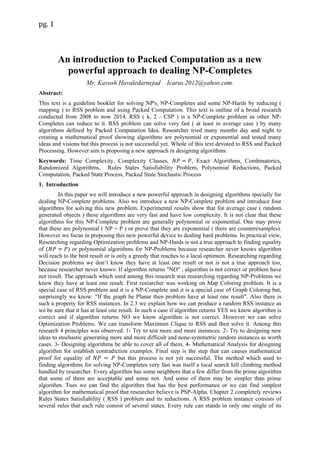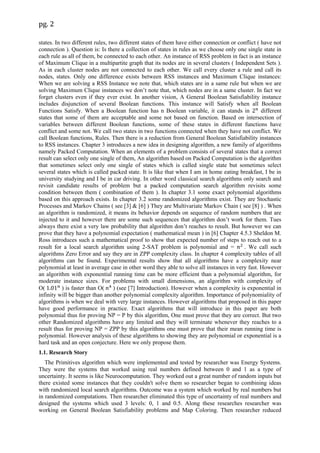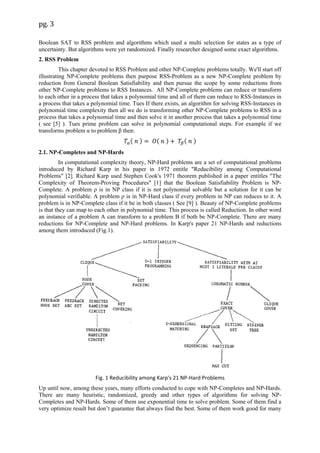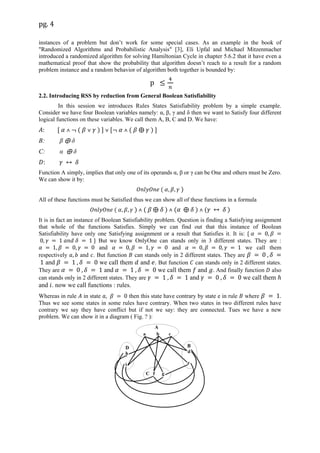Anúncio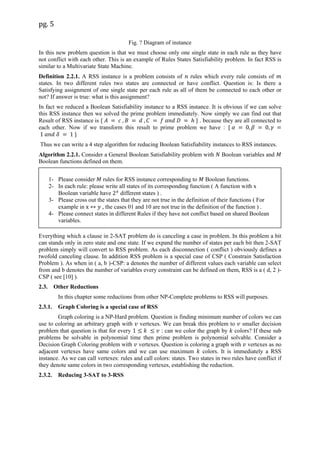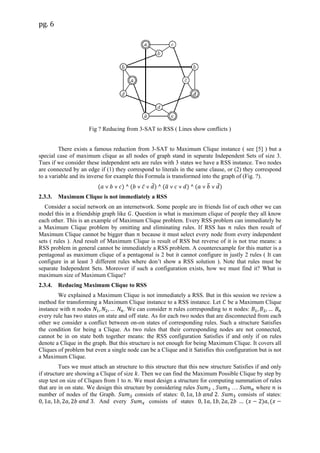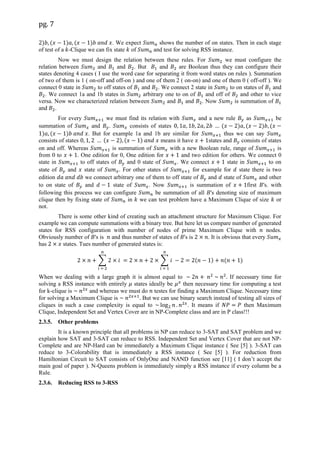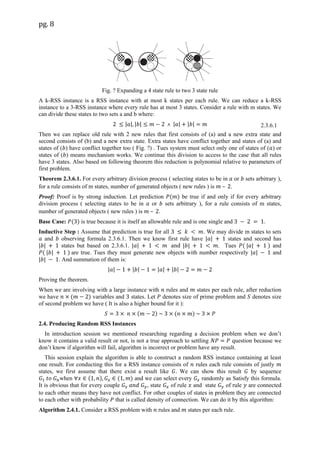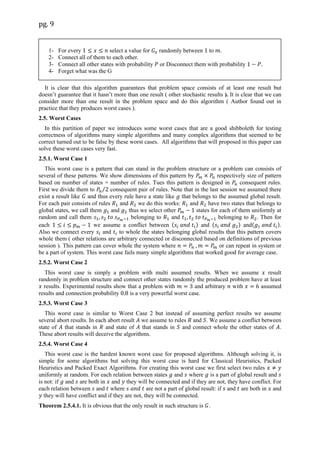Anúncio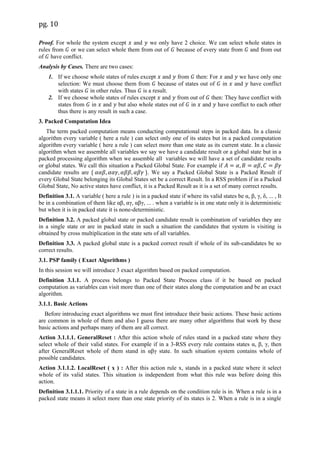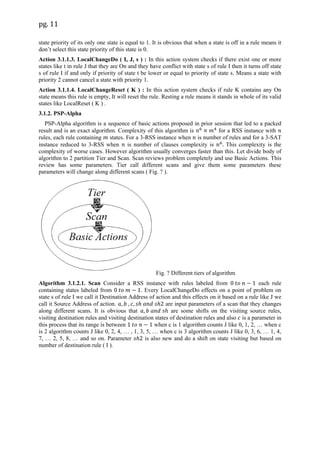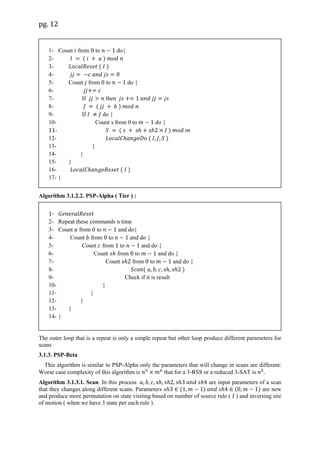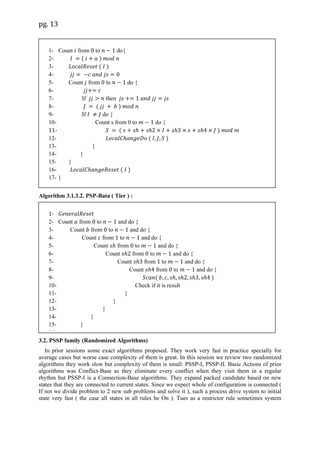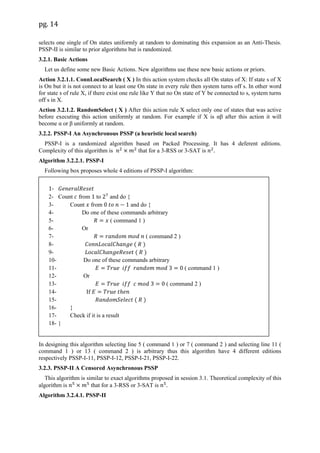Anúncio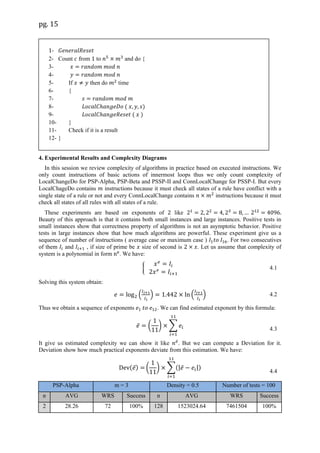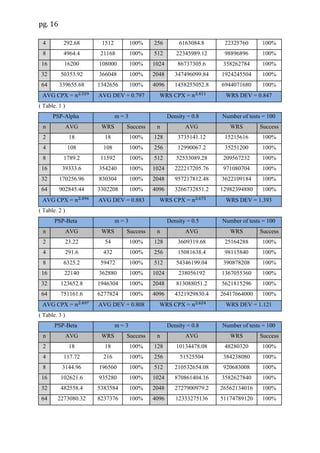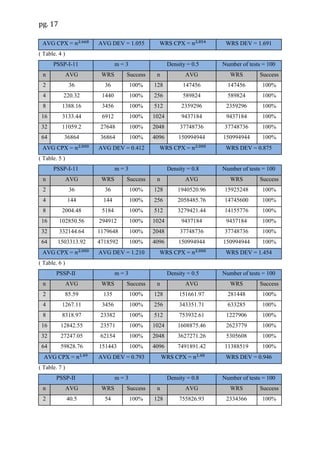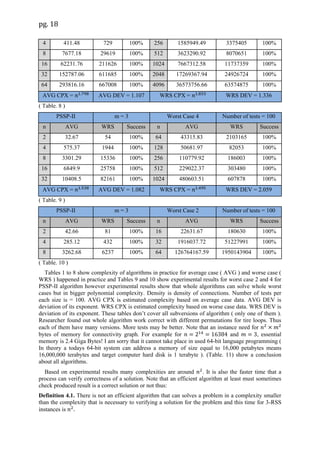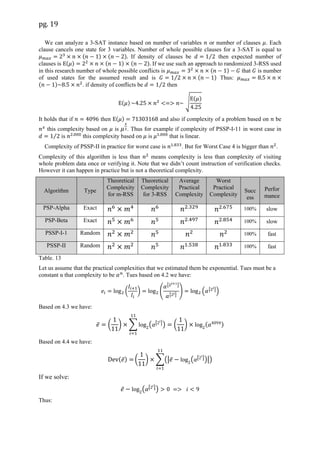Anúncio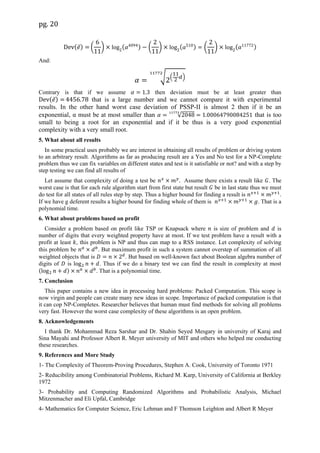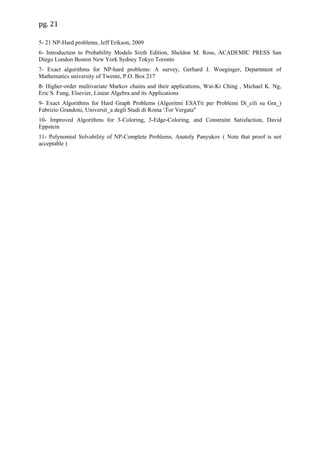Próximos SlideShares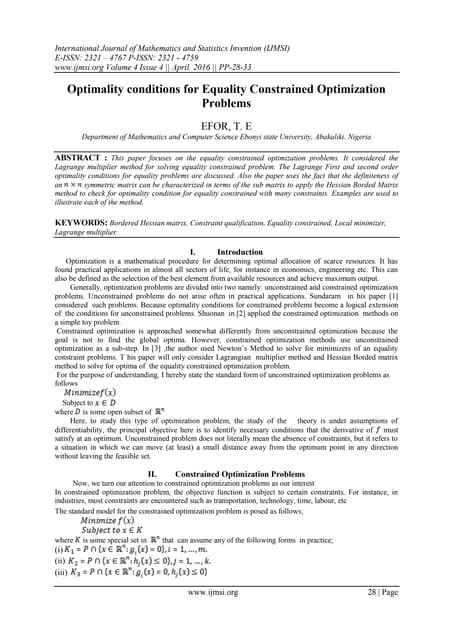Optimality conditions for Equality Constrained Optimization Problems
Carregando em ... 3
1 de 21
Anúncio

### OCT-20

2. pg. 2 states. In two different rules, two different states of them have either connection or conflict ( have not connection ). Question is: Is there a collection of states in rules as we choose only one single state in each rule as all of them, be connected to each other. An instance of RSS problem in fact is an instance of Maximum Clique in a multipartite graph that its nodes are in several clusters ( Independent Sets ). As in each cluster nodes are not connected to each other. We call every cluster a rule and call its nodes, states. Only one difference exists between RSS instances and Maximum Clique instances: When we are solving a RSS Instance we note that, which states are in a same rule but when we are solving Maximum Clique instances we don’t note that, which nodes are in a same cluster. In fact we forget clusters even if they ever exist. In another vision, A General Boolean Satisfiability instance includes disjunction of several Boolean functions. This instance will Satisfy when all Boolean Functions Satisfy. When a Boolean function has n Boolean variable, it can stands in different states that some of them are acceptable and some not based on function. Based on intersection of variables between different Boolean functions, some of these states in different functions have conflict and some not. We call two states in two functions connected when they have not conflict. We call Boolean functions, Rules. Then there is a reduction from General Boolean Satisfiability instances to RSS instances. Chapter 3 introduces a new idea in designing algorithm, a new family of algorithms namely Packed Computation. When an elements of a problem consists of several states that a correct result can select only one single of them, An algorithm based on Packed Computation is the algorithm that sometimes select only one single of states which is called single state but sometimes select several states which is called packed state. It is like that when I am in home eating breakfast, I be in university studying and I be in car driving. In other word classical search algorithms only search and revisit candidate results of problem but a packed computation search algorithm revisits some condition between them ( combination of them ). In chapter 3.1 some exact polynomial algorithms based on this approach exists. In chapter 3.2 some randomized algorithms exist. They are Stochastic Processes and Markov Chains ( see  &  ) They are Multivariate Markov Chain ( see  ) . When an algorithm is randomized, it means its behavior depends on sequence of random numbers that are injected to it and however there are some such sequences that algorithm don’t work for them. Tues always there exist a very law probability that algorithm don’t reaches to result. But however we can prove that they have a polynomial expectation ( mathematical mean ) in  Chapter 4.5.3 Sheldon M. Ross introduces such a mathematical proof to show that expected number of steps to reach out to a result for a local search algorithm using 2-SAT problem is polynomial and = . We call such algorithms Zero Error and say they are in ZPP complexity class. In chapter 4 complexity tables of all algorithms can be found. Experimental results show that all algorithms have a complexity near polynomial at least in average case in other word they able to solve all instances in very fast. However an algorithm with exponential running time can be more efficient than a polynomial algorithm, for moderate instance sizes. For problems with small dimensions, an algorithm with complexity of O( ) is faster than O( ) (see  Introduction). However when a complexity is exponential in infinity will be bigger than another polynomial complexity algorithm. Importance of polynomiality of algorithms is when we deal with very large instances. However algorithms that proposed in this paper have good performance in practice. Exact algorithms that will introduce in this paper are both polynomial thus for proving NP = P by this algorithm, One must prove that they are correct. But two other Randomized algorithms have any limited and they will terminate whenever they reaches to a result thus for proving NP = ZPP by this algorithms one must prove that their mean running time is polynomial. However analysis of these algorithms to showing they are polynomial or exponential is a hard task and an open conjecture. Here we only propose them. 1.1. Research Story The Primitives algorithm which were implemented and tested by researcher was Energy Systems. They were the systems that worked using real numbers defined between 0 and 1 as a type of uncertainty. It seems is like Neurocomputation. They worked out a great number of random inputs but there existed some instances that they couldn't solve them so researcher began to combining ideas with randomized local search algorithms. Outcome was a system which worked by real numbers but in randomized computations. Then researcher eliminated this type of uncertainty of real numbers and designed the systems which used 3 levels: 0, 1 and 0.5. Along these researches researcher was working on General Boolean Satisfiability problems and Map Coloring. Then researcher reduced
3. pg. 3 Boolean SAT to RSS problem and algorithms which used a multi selection for states as a type of uncertainty. But algorithms were yet randomized. Finally researcher designed some exact algorithms. 2. RSS Problem This chapter devoted to RSS Problem and other NP-Complete problems totally. We'll start off illustrating NP-Complete problems then purpose RSS-Problem as a new NP-Complete problem by reduction from General Boolean Satisfiability and then pursue the scope by some reductions from other NP-Complete problems to RSS Instances. All NP-Complete problems can reduce or transform to each other in a process that takes a polynomial time and all of them can reduce to RSS-Instances in a process that takes a polynomial time. Tues If there exists, an algorithm for solving RSS-Instances in polynomial time complexity then all we do is transforming other NP-Complete problems to RSS in a process that takes a polynomial time and then solve it in another process that takes a polynomial time ( see  ). Tues prime problem can solve in polynomial computational steps. For example if we transforms problem α to problem β then: ) ) ) 2.1. NP-Completes and NP-Hards In computational complexity theory, NP-Hard problems are a set of computational problems introduced by Richard Karp in his paper in 1972 entitle "Reducibility among Computational Problems" . Richard Karp used Stephen Cook's 1971 theorem published in a paper entitles "The Complexity of Theorem-Proving Procedures"  that the Boolean Satisfiability Problem is NP- Complete. A problem ρ is in NP class if it is not polynomial solvable but a solution for it can be polynomial verifiable. A problem ρ is in NP-Hard class if every problem in NP can reduces to it. A problem is in NP-Complete class if it be in both classes ( See  ). Beauty of NP-Complete problems is that they can map to each other in polynomial time. This process is called Reduction. In other word an instance of a problem A can transform to a problem B if both be NP-Complete. There are many reductions for NP-Complete and NP-Hard problems. In Karp's paper 21 NP-Hards and reductions among them introduced (Fig.1). Fig. 1 Reducibility among Karp's 21 NP-Hard Problems Up until now, among these years, many efforts conducted to cope with NP-Completes and NP-Hards. There are many heuristic, randomized, greedy and other types of algorithms for solving NP- Completes and NP-Hards. Some of them use exponential time to solve problem. Some of them find a very optimize result but don’t guarantee that always find the best. Some of them work good for many
4. pg. 4 instances of a problem but don’t work for some special cases. As an example in the book of "Randomized Algorithms and Probabilistic Analysis" , Eli Upfal and Michael Mitzenmacher introduced a randomized algorithm for solving Hamiltonian Cycle in chapter 5.6.2 that it have even a mathematical proof that show the probability that algorithm doesn’t reach to a result for a random problem instance and a random behavior of algorithm both together is bounded by: 2.2. Introducing RSS by reduction from General Boolean Satisfiability In this session we introduces Rules States Satisfiability problem by a simple example. Consider we have four Boolean variables namely: α, β, γ and δ then we want to Satisfy four different logical functions on these variables. We call them A, B, C and D. We have: ) ) B: β δ C: α δ Function A simply, implies that only one of its operands α, β or γ can be One and others must be Zero. We can show it by: ) All of these functions must be Satisfied thus we can show all of these functions in a formula ) ) ) ) It is in fact an instance of Boolean Satisfiability problem. Question is finding a Satisfying assignment that whole of the functions Satisfies. Simply we can find out that this instance of Boolean Satisfiability have only one Satisfying assignment or a result that Satisfies it. It is: But we know OnlyOne can stands only in 3 different states. They are : and and we call them respectively and . But function can stands only in 2 different states. They are and we call them and . But function can stands only in 2 different states. They are and we call them and . And finally function also can stands only in 2 different states. They are and we call them and . now we call functions : rules. Whereas in rule in state then this state have contrary by state e in rule where . Thus we see some states in some rules have contrary. When two states in two different rules have contrary we say they have conflict but if not we say: they are connected. Tues we have a new problem. We can show it in a diagram ( Fig. ? ):
7. pg. 7 ) ) ) . We expect shows the number of on states. Then in each stage of test of a -Clique we can fix state of and test for solving RSS instance. Now we must design the relation between these rules. For we must configure the relation between and and . But and are Boolean thus they can configure their states denoting 4 cases ( I use the word case for separating it from word states on rules ). Summation of two of them is 1 ( on-off and off-on ) and one of them 2 ( on-on) and one of them 0 ( off-off ). We connect 0 state in to off states of and . We connect 2 state in to on states of and . We connect 1a and 1b states in arbitrary one to on of and off of and other to vice versa. Now we characterized relation between and and . Now is summation of and . For every we must find its relation with and a new rule as be summation of and . consists of states ) ) ) ) . But for example 1a and 1b are similar for thus we can say consists of states ) ) means it have states and consists of states on and off. Whereas is summation of with a new Boolean rule, range of is from 0 to . One edition for 0, One edition for and two edition for others. We connect 0 state in to off states of and 0 state of . We connect state in to on state of and state of . For other states of for example for state there is two edition we connect arbitrary one of them to off state of and state of and other to on state of and state of . Now is summation of first 's. with following this process we can configure be summation of all 's denoting size of maximum clique then by fixing state of in we can test problem have a Maximum Clique of size or not. There is some other kind of creating such an attachment structure for Maximum Clique. For example we can compute summations with a binary tree. But here let us compare number of generated states for RSS configuration with number of nodes of prime Maximum Clique with nodes. Obviously number of 's is and thus number of states of 's is . It is obvious that every has states. Tues number of generated states is: ∑ ∑ ) ) When we dealing with a large graph it is almost equal to If necessary time for solving a RSS instance with entirely states ideally be then necessary time for computing a test for k-clique is and whereas we must do testes for finding a Maximum Clique. Necessary time for solving a Maximum Clique is But we can use binary search instead of testing all sizes of cliques in such a case complexity is equal to . It means if then Maximum Clique, Independent Set and Vertex Cover are in NP-Complete class and are in P class!!! 2.3.5. Other problems It is a known principle that all problems in NP can reduce to 3-SAT and SAT problem and we explain how SAT and 3-SAT can reduce to RSS. Independent Set and Vertex Cover that are not NP- Complete and are NP-Hard can be immediately a Maximum Clique instance ( See  ). 3-SAT can reduce to 3-Colorability that is immediately a RSS instance ( See  ). For reduction from Hamiltonian Circuit to SAT consists of OnlyOne and NAND function see  ( I don’t accept the main goal of paper ). N-Queens problem is immediately simply a RSS instance if every column be a Rule. 2.3.6. Reducing RSS to 3-RSS
8. pg. 8 Fig. ? Expanding a 4 state rule to two 3 state rule A k-RSS instance is a RSS instance with at most k states per each rule. We can reduce a k-RSS instance to a 3-RSS instance where every rule has at most 3 states. Consider a rule with m states. We can divide these states to two sets a and b where: | | | | | | | | Then we can replace old rule with 2 new rules that first consists of (a) and a new extra state and second consists of (b) and a new extra state. Extra states have conflict together and states of (a) and states of ( ) have conflict together too ( Fig. ?) . Tues system must select only one of states of ( ) or states of ( ) means mechanism works. We continue this division to access to the case that all rules have 3 states. Also based on following theorem this reduction is polynomial relative to parameters of first problem. Theorem 2.3.6.1. For every arbitrary division process ( selecting states to be in or sets arbitrary ), for a rule consists of m states, number of generated objects ( new rules ) is – . Proof: Proof is by strong induction. Let prediction ) be true if and only if for every arbitrary division process ( selecting states to be in or sets arbitrary ), for a rule consists of m states, number of generated objects ( new rules ) is – . Base Case: ) is true because it is itself an allowable rule and is one single and . Inductive Step : Assume that prediction is true for all . We may divide m states to sets and observing formula 2.3.6.1. Then we know first rule have | | states and second has | | states but based on 2.3.6.1. | | and | | . Tues | | ) and | | ) are true. Tues they must generate new objects with number respectively | | and | | . And summation of them is: | | | | | | | | Proving the theorem. When we are involving with a large instance with rules and states per each rule, after reduction we have ) variables and states. Let denotes size of prime problem and denotes size of second problem we have ( It is also a higher bound for it ): ) ) 2.4. Producing Random RSS Instances In introduction session we mentioned researching regarding a decision problem when we don’t know it contains a valid result or not, is not a true approach to settling question because we don’t know if algorithm will fail, algorithm is incorrect or problem have any result. This session explain the algorithm is able to construct a random RSS instance containing at least one result. For conducting this for a RSS instance consists of rules each rule consists of justly states, we first assume that there exist a result like We can show this result by sequence when ) ) and we can select every randomly as Satisfy this formula. It is obvious that for every couple , state of rule and state of rule are connected to each other means they have not conflict. For other couples of states in problem they are connected to each other with probability that is called density of connection. We can do it by this algorithm: Algorithm 2.4.1. Consider a RSS problem with rules and states per each rule. 2.3.6.1
9. pg. 9 It is clear that this algorithm guarantees that problem space consists of at least one result but doesn’t guarantee that it hasn’t more than one result ( other stochastic results ). It is clear that we can consider more than one result in the problem space and do this algorithm ( Author found out in practice that they produces worst cases ). 2.5. Worst Cases In this partition of paper we introduces some worst cases that are a good shibboleth for testing correctness of algorithms many simple algorithms and many complex algorithms that seemed to be correct turned out to be false by these worst cases. All algorithms that will proposed in this paper can solve these worst cases very fast. 2.5.1. Worst Case 1 This worst case is a pattern that can stand in the problem structure or a problem can consists of several of these patterns. We show dimensions of this pattern by respectively size of pattern based on number of states × number of rules. Tues this pattern is designed in consequent rules. First we divide them to ⁄ consequent pair of rules. Note that in the last session we assumed there exist a result like and thus every rule have a state like that belongs to the assumed global result. For each pair consists of rules and we do this works: and have two states that belongs to global states, we call them and thus we select other states for each of them uniformly at random and call them belonging to and belonging to . Then for each we assume a conflict between ) and ) and ). Also we connect every and to whole the states belonging global results that this pattern covers whole them ( other relations are arbitrary connected or disconnected based on definitions of previous session ). This pattern can cover whole the system where or can repeat in system or be a part of system. This worst case fails many simple algorithms that worked good for average case. 2.5.2. Worst Case 2 This worst case is simply a problem with multi assumed results. When we assume result randomly in problem structure and connect other states randomly the produced problem have at least results. Experimental results show that a problem with and arbitrary with assumed results and connection probability is a very powerful worst case. 2.5.3. Worst Case 3 This worst case is similar to Worst Case 2 but instead of assuming perfect results we assume several abort results. In each abort result we assume to rules and . We assume a conflict between state of that stands in and state of that stands in and connect whole the other states of . These abort results will deceive the algorithms. 2.5.4. Worst Case 4 This worst case is the hardest known worst case for proposed algorithms. Although solving it, is simple for some algorithms but solving this worst case is hard for Classical Heuristics, Packed Heuristics and Packed Exact Algorithms. For creating this worst case we first select two rules uniformly at random. For each relation between states and where is a part of global result and is not: if and are both in and they will be connected and if they are not, they have conflict. For each relation between and where are not a part of global result: if and are both in and they will have conflict and if they are not, they will be connected. Theorem 2.5.4.1. It is obvious that the only result in such structure is . 1- For every 𝑥 𝑛 select a value for 𝐺 𝑥 randomly between to 𝑚 2- Connect all of them to each other. 3- Connect all other states with probability 𝑃 or Disconnect them with probability 𝑃 4- Forget what was the G
10. pg. 10 Proof. For whole the system except and we only have 2 choice. We can select whole states in rules from or we can select whole them from out of because of every state from and from out of have conflict. Analysis by Cases. There are two cases: 1. If we choose whole states of rules except and from then: For and we have only one selection: We must choose them from because of states out of in and have conflict with states in other rules. Thus is a result. 2. If we choose whole states of rules except and from out of then: They have conflict with states from in and but also whole states out of in and have conflict to each other thus there is any result in such a case. 3. Packed Computation Idea The term packed computation means conducting computational steps in packed data. In a classic algorithm every variable ( here a rule ) can select only one of its states but in a packed computation algorithm every variable ( here a rule ) can select more than one state as its current state. In a classic algorithm when we assemble all variables we say we have a candidate result or a global state but in a packed processing algorithm when we assemble all variables we will have a set of candidate results or global states. We call this situation a Packed Global State. For example if candidate results are . We say a Packed Global State is a Packed Result if every Global State belonging its Global States set be a correct Result. In a RSS problem if in a Packed Global State, No active states have conflict, it is a Packed Result as it is a set of many correct results. Definition 3.1. A variable ( here a rule ) is in a packed state if where its valid states be α, β, γ, δ, ... , It be in a combination of them like αβ, αγ, αβγ, ... . when a variable is in one state only it is deterministic but when it is in packed state it is none-deterministic. Definition 3.2. A packed global state or packed candidate result is combination of variables they are in a single state or are in packed state in such a situation the candidates that system is visiting is obtained by cross multiplication in the state sets of all variables. Definition 3.3. A packed global state is a packed correct result if whole of its sub-candidates be so correct results. 3.1. PSP family ( Exact Algorithms ) In this session we will introduce 3 exact algorithm based on packed computation. Definition 3.1.1. A process belongs to Packed State Process class if it be based on packed computation as variables can visit more than one of their states along the computation and be an exact algorithm. 3.1.1. Basic Actions Before introducing exact algorithms we must first introduce their basic actions. These basic actions are common in whole of them and also I guess there are many other algorithms that work by these basic actions and perhaps many of them are all correct. Action 3.1.1.1. GeneralReset : After this action whole of rules stand in a packed state where they select whole of their valid states. For example if in a 3-RSS every rule contains states α, β, γ, then after GeneralReset whole of them stand in αβγ state. In such situation system contains whole of possible candidates. Action 3.1.1.2. LocalReset ( x ) : After this action rule x, stands in a packed state where it select whole of its valid states. This situation is independent from what this rule was before doing this action. Definition 3.1.1.1. Priority of a state in a rule depends on the condition rule is in. When a rule is in a packed state means it select more than one state priority of its states is 2. When a rule is in a single
11. pg. 11 state priority of its only one state is equal to 1. It is obvious that when a state is off in a rule means it don’t select this state priority of this state is 0. Action 3.1.1.3. LocalChangeDo ( I, J, s ) : In this action system checks if there exist one or more states like t in rule J that they are On and they have conflict with state s of rule I then it turns off state s of rule I if and only if priority of state t be lower or equal to priority of state s. Means a state with priority 2 cannot cancel a state with priority 1. Action 3.1.1.4. LocalChangeReset ( K ) : In this action system checks if rule K contains any On state means this rule is empty, It will reset the rule. Resting a rule means it stands in whole of its valid states like LocalReset ( K ) . 3.1.2. PSP-Alpha PSP-Alpha algorithm is a sequence of basic actions proposed in prior session that led to a packed result and is an exact algorithm. Complexity of this algorithm is for a RSS instance with rules, each rule containing states. For a 3-RSS instance when is number of rules and for a 3-SAT instance reduced to 3-RSS when is number of clauses complexity is This complexity is the complexity of worse cases. However algorithm usually converges faster than this. Let divide body of algorithm to 2 partition Tier and Scan. Scan reviews problem completely and use Basic Actions. This review has some parameters. Tier call different scans and give them some parameters these parameters will change along different scans ( Fig. ? ). Fig. ? Different tiers of algorithm Algorithm 3.1.2.1. Scan Consider a RSS instance with rules labeled from each rule containing states labeled from . Every LocalChangeDo effects on a point of problem on state s of rule I we call it Destination Address of action and this effects on it based on a rule like J we call it Source Address of action. are input parameters of a scan that they changes along different scans. It is obvious that are some shifts on the visiting source rules, visiting destination rules and visiting destination states of destination rules and also is a parameter in this process that its range is between when c is 1 algorithm counts J like 0, 1, 2, … when c is 2 algorithm counts J like 0, 2, 4, … , 1, 3, 5, … when c is 3 algorithm counts J like 0, 3, 6, … 1, 4, 7, … 2, 5, 8, … and so on. Parameter is also new and do a shift on state visiting but based on number of destination rule ( I ).
12. pg. 12 Algorithm 3.1.2.2. PSP-Alpha ( Tier ) : The outer loop that is a repeat is only a simple repeat but other loop produce different parameters for scans 3.1.3. PSP-Beta This algorithm is similar to PSP-Alpha only the parameters that will change in scans are different: Worse case complexity of this algorithm is that for a 3-RSS or a reduced 3-SAT is Algorithm 3.1.3.1. Scan. In this process are input parameters of a scan that they changes along different scans. Parameters ) ) are new and produce more permutation on state visiting based on number of source rule ( J ) and inversing site of motion ( when we have 3 state per each rule ). 1- Count 𝑖 from to 𝑛 do{ 2- 𝐼 𝑖 𝑎 ) 𝑚𝑜𝑑 𝑛 3- 𝐿𝑜𝑐𝑎𝑙𝑅𝑒𝑠𝑒𝑡 𝐼 ) 4- 𝑗𝑗 𝑐 𝑎𝑛𝑑 𝑗𝑠 5- Count 𝑗 from to 𝑛 do { 6- 𝑗𝑗 𝑐 7- If 𝑗𝑗 > 𝑛 then 𝑗𝑠 𝑎𝑛𝑑 𝑗𝑗 𝑗𝑠 8- 𝐽 𝑗𝑗 𝑏 ) 𝑚𝑜𝑑 𝑛 9- If 𝐼 𝐽 do { 10- Count s from 0 to 𝑚 do { 11- 𝑆 𝑠 𝑠 𝑠 𝐼 ) 𝑚𝑜𝑑 𝑚 12- 𝐿𝑜𝑐𝑎𝑙𝐶 𝑎𝑛𝑔𝑒𝐷𝑜 𝐼 𝐽 𝑆 ) 13- } 14- } 15- } 16- 𝐿𝑜𝑐𝑎𝑙𝐶 𝑎𝑛𝑔𝑒𝑅𝑒𝑠𝑒𝑡 𝐼 ) 17- } 1- 𝐺𝑒𝑛𝑒𝑟𝑎𝑙𝑅𝑒𝑠𝑒𝑡 2- Repeat these commands n time 3- Count 𝑎 from to 𝑛 and do{ 4- Count 𝑏 from to 𝑛 and do { 5- Count 𝑐 from to 𝑛 and do { 6- Count 𝑠 from to 𝑚 and do { 7- Count 𝑠 from to 𝑚 and do { 8- 𝑆𝑐𝑎𝑛 𝑎 𝑏 𝑐 𝑠 𝑠 ) 9- Check if it is result 10- } 11- } 12- } 13- } 14- }
13. pg. 13 Algorithm 3.1.3.2. PSP-Bata ( Tier ) : 3.2. PSSP family (Randomized Algorithms) In prior sessions some exact algorithms proposed. They work very fast in practice specially for average cases but worse case complexity of them is great. In this session we review two randomized algorithms they work slow but complexity of them is small: PSSP-I, PSSP-II. Basic Actions of prior algorithms was Conflict-Base as they eliminate every conflict when they visit them in a regular rhythm but PSSP-I is a Connection-Base algorithms. They expand packed candidate based on new states that they are connected to current states. Since we expect whole of configuration is connected ( If not we divide problem to 2 new sub problems and solve it ), such a process drive system to initial state very fast ( the case all states in all rules be On ). Tues as a restrictor rule sometimes system 1- Count 𝑖 from to 𝑛 do{ 2- 𝐼 𝑖 𝑎 ) 𝑚𝑜𝑑 𝑛 3- 𝐿𝑜𝑐𝑎𝑙𝑅𝑒𝑠𝑒𝑡 𝐼 ) 4- 𝑗𝑗 𝑐 𝑎𝑛𝑑 𝑗𝑠 5- Count 𝑗 from to 𝑛 do { 6- 𝑗𝑗 𝑐 7- If 𝑗𝑗 > 𝑛 then 𝑗𝑠 𝑎𝑛𝑑 𝑗𝑗 𝑗𝑠 8- 𝐽 𝑗𝑗 𝑏 ) 𝑚𝑜𝑑 𝑛 9- If 𝐼 𝐽 do { 10- Count s from 0 to 𝑚 do { 11- 𝑆 𝑠 𝑠 𝑠 𝐼 𝑠 𝑠 𝑠 𝐽 ) 𝑚𝑜𝑑 𝑚 12- 𝐿𝑜𝑐𝑎𝑙𝐶 𝑎𝑛𝑔𝑒𝐷𝑜 𝐼 𝐽 𝑆 ) 13- } 14- } 15- } 16- 𝐿𝑜𝑐𝑎𝑙𝐶 𝑎𝑛𝑔𝑒𝑅𝑒𝑠𝑒𝑡 𝐼 ) 17- } 1- 𝐺𝑒𝑛𝑒𝑟𝑎𝑙𝑅𝑒𝑠𝑒𝑡 2- Count 𝑎 from to 𝑛 and do { 3- Count 𝑏 from to 𝑛 and do { 4- Count 𝑐 from to 𝑛 and do { 5- Count 𝑠 from to 𝑚 and do { 6- Count 𝑠 from to 𝑚 and do { 7- Count 𝑠 from to 𝑚 and do { 8- Count 𝑠 from to 𝑚 and do { 9- 𝑆𝑐𝑎𝑛 𝑏 𝑐 𝑠 𝑠 𝑠 𝑠 ) 10- Check if it is result 11- } 12- } 13- } 14- } 15- } 16- }
14. pg. 14 selects one single of On states uniformly at random to dominating this expansion as an Anti-Thesis. PSSP-II is similar to prior algorithms but is randomized. 3.2.1. Basic Actions Let us define some new Basic Actions. New algorithms use these new basic actions or priors. Action 3.2.1.1. ConnLocalSearch ( X ) In this action system checks all On states of X: If state s of X is On but it is not connect to at least one On state in every rule then system turns off s. In other word for state s of rule X, if there exist one rule like Y that no On state of Y be connected to s, system turns off s in X. Action 3.2.1.2. RandomSelect ( X ) After this action rule X select only one of states that was active before executing this action uniformly at random. For example if X is αβ after this action it will become α or β uniformly at random. 3.2.2. PSSP-I An Asynchronous PSSP (a heuristic local search) PSSP-I is a randomized algorithm based on Packed Processing. It has 4 deferent editions. Complexity of this algorithm is that for a 3-RSS or 3-SAT is Algorithm 3.2.2.1. PSSP-I Following box proposes whole 4 editions of PSSP-I algorithm: In designing this algorithm selecting line 5 ( command 1 ) or 7 ( command 2 ) and selecting line 11 ( command 1 ) or 13 ( command 2 ) is arbitrary thus this algorithm have 4 different editions respectively PSSP-I-11, PSSP-I-12, PSSP-I-21, PSSP-I-22. 3.2.3. PSSP-II A Censored Asynchronous PSSP This algorithm is similar to exact algorithms proposed in session 3.1. Theoretical complexity of this algorithm is that for a 3-RSS or 3-SAT is Algorithm 3.2.4.1. PSSP-II 1- 𝐺𝑒𝑛𝑒𝑟𝑎𝑙𝑅𝑒𝑠𝑒𝑡 2- Count 𝑐 from to 7 and do { 3- Count 𝑥 from 𝑡𝑜 𝑛 and do { 4- Do one of these commands arbitrary 5- 𝑅 𝑥 ( command 1 ) 6- Or 7- 𝑅 𝑟𝑎𝑛𝑑𝑜𝑚 𝑚𝑜𝑑 𝑛 ( command 2 ) 8- 𝐶𝑜𝑛𝑛𝐿𝑜𝑐𝑎𝑙𝐶 𝑎𝑛𝑔𝑒 𝑅 ) 9- 𝐿𝑜𝑐𝑎𝑙𝐶 𝑎𝑛𝑔𝑒𝑅𝑒𝑠𝑒𝑡 𝑅 ) 10- Do one of these commands arbitrary 11- 𝐸 𝑇𝑟𝑢𝑒 𝑖𝑓𝑓 𝑟𝑎𝑛𝑑𝑜𝑚 𝑚𝑜𝑑 ( command 1 ) 12- Or 13- 𝐸 𝑇𝑟𝑢𝑒 𝑖𝑓𝑓 𝑐 𝑚𝑜𝑑 ( command 2 ) 14- If 𝐸 𝑇𝑟𝑢𝑒 𝑡 𝑒𝑛 15- 𝑅𝑎𝑛𝑑𝑜𝑚𝑆𝑒𝑙𝑒𝑐𝑡 𝑅 ) 16- } 17- Check if it is a result 18- }
15. pg. 15 4. Experimental Results and Complexity Diagrams In this session we review complexity of algorithms in practice based on executed instructions. We only count instructions of basic actions of innermost loops thus we only count complexity of LocalChangeDo for PSP-Alpha, PSP-Beta and PSSP-II and ConnLocalChange for PSSP-I. But every LocalChageDo contains instructions because it must check all states of a rule have conflict with a single state of a rule or not and every ConnLocalChange contains instructions because it must check all states of all rules with all states of a rule. These experiments are based on exponents of like Beauty of this approach is that it contains both small instances and large instances. Positive tests in small instances show that correctness property of algorithms is not an asymptotic behavior. Positive tests in large instances show that how much algorithms are powerful. These experiment give us a sequence of number of instructions ( average case or maximum case ) . For two consecutives of them and , if size of prime be size of second is . Let us assume that complexity of system is a polynomial in form . We have: { Solving this system obtain: ( ) ( ) Thus we obtain a sequence of exponents . We can find estimated exponent by this formula: ̅ ( ) ∑ It give us estimated complexity we can show it like ̅ . But we can compute a Deviation for it. Deviation show how much practical exponents deviate from this estimation. We have: ̅) ( ) ∑ | ̅ |) PSP-Alpha m = 3 Density = 0.5 Number of tests = 100 n AVG WRS Success n AVG WRS Success 2 28.26 72 100% 128 1523024.64 7461504 100% 1- 𝐺𝑒𝑛𝑒𝑟𝑎𝑙𝑅𝑒𝑠𝑒𝑡 2- Count 𝑐 from to 𝑛 𝑚 and do { 3- 𝑥 𝑟𝑎𝑛𝑑𝑜𝑚 𝑚𝑜𝑑 𝑛 4- 𝑦 𝑟𝑎𝑛𝑑𝑜𝑚 𝑚𝑜𝑑 𝑛 5- If 𝑥 𝑦 then do 𝑚 time 6- { 7- 𝑠 𝑟𝑎𝑛𝑑𝑜𝑚 𝑚𝑜𝑑 𝑚 8- 𝐿𝑜𝑐𝑎𝑙𝐶 𝑎𝑛𝑔𝑒𝐷𝑜 𝑥 𝑦 𝑠) 9- 𝐿𝑜𝑐𝑎𝑙𝐶 𝑎𝑛𝑔𝑒𝑅𝑒𝑠𝑒𝑡 𝑥 ) 10- } 11- Check if it is a result 12- } 4.1 4.2 4.3 4.4
16. pg. 16 4 292.68 1512 100% 256 6163084.8 22325760 100% 8 4964.4 21168 100% 512 22345989.12 98896896 100% 16 16200 108000 100% 1024 86737305.6 358262784 100% 32 50353.92 366048 100% 2048 347496099.84 1924245504 100% 64 339655.68 1342656 100% 4096 1458255052.8 6944071680 100% AVG CPX = AVG DEV = 0.797 WRS CPX = WRS DEV = 0.847 ( Table. 1 ) PSP-Alpha m = 3 Density = 0.8 Number of tests = 100 n AVG WRS Success n AVG WRS Success 2 18 18 100% 128 3735141.12 15215616 100% 4 108 108 100% 256 12990067.2 35251200 100% 8 1789.2 11592 100% 512 52533089.28 209567232 100% 16 39333.6 354240 100% 1024 222217205.76 971080704 100% 32 170256.96 830304 100% 2048 957217812.48 3622109184 100% 64 902845.44 3302208 100% 4096 3266732851.2 12982394880 100% AVG CPX = AVG DEV = 0.883 WRS CPX = 7 WRS DEV = 1.393 ( Table. 2 ) PSP-Beta m = 3 Density = 0.5 Number of tests = 100 n AVG WRS Success n AVG WRS Success 2 23.22 54 100% 128 3609319.68 25164288 100% 4 291.6 432 100% 256 15081638.4 98115840 100% 8 6325.2 59472 100% 512 54346199.04 390878208 100% 16 22140 362880 100% 1024 238056192 1367055360 100% 32 123652.8 1946304 100% 2048 813088051.2 5621815296 100% 64 751161.6 6277824 100% 4096 4321929830.4 26417664000 100% AVG CPX = 7 AVG DEV = 0.808 WRS CPX = WRS DEV = 1.121 ( Table. 3 ) PSP-Beta m = 3 Density = 0.8 Number of tests = 100 n AVG WRS Success n AVG WRS Success 2 18 18 100% 128 10134478.08 48280320 100% 4 117.72 216 100% 256 51525504 384238080 100% 8 3144.96 196560 100% 512 210532654.08 920683008 100% 16 102621.6 935280 100% 1024 870861404.16 3582627840 100% 32 482558.4 5383584 100% 2048 2727900979.2 26562134016 100% 64 2273080.32 8237376 100% 4096 12333275136 51174789120 100%
17. pg. 17 AVG CPX = AVG DEV = 1.055 WRS CPX = WRS DEV = 1.691 ( Table. 4 ) PSSP-I-11 m = 3 Density = 0.5 Number of tests = 100 n AVG WRS Success n AVG WRS Success 2 36 36 100% 128 147456 147456 100% 4 220.32 1440 100% 256 589824 589824 100% 8 1388.16 3456 100% 512 2359296 2359296 100% 16 3133.44 6912 100% 1024 9437184 9437184 100% 32 11059.2 27648 100% 2048 37748736 37748736 100% 64 36864 36864 100% 4096 150994944 150994944 100% AVG CPX = AVG DEV = 0.412 WRS CPX = WRS DEV = 0.875 ( Table. 5 ) PSSP-I-11 m = 3 Density = 0.8 Number of tests = 100 n AVG WRS Success n AVG WRS Success 2 36 36 100% 128 1940520.96 15925248 100% 4 144 144 100% 256 2058485.76 14745600 100% 8 2004.48 5184 100% 512 3279421.44 14155776 100% 16 102850.56 294912 100% 1024 9437184 9437184 100% 32 332144.64 1179648 100% 2048 37748736 37748736 100% 64 1503313.92 4718592 100% 4096 150994944 150994944 100% AVG CPX = AVG DEV = 1.210 WRS CPX = WRS DEV = 1.454 ( Table. 6 ) PSSP-II m = 3 Density = 0.5 Number of tests = 100 n AVG WRS Success n AVG WRS Success 2 85.59 135 100% 128 151661.97 281448 100% 4 1267.11 3456 100% 256 343351.71 633285 100% 8 8318.97 23382 100% 512 753932.61 1227906 100% 16 12842.55 23571 100% 1024 1608875.46 2623779 100% 32 27247.05 62154 100% 2048 3627271.26 5305608 100% 64 59828.76 151443 100% 4096 7491891.42 11388519 100% AVG CPX = AVG DEV = 0.793 WRS CPX = WRS DEV = 0.946 ( Table. 7 ) PSSP-II m = 3 Density = 0.8 Number of tests = 100 n AVG WRS Success n AVG WRS Success 2 40.5 54 100% 128 755826.93 2334366 100%
18. pg. 18 4 411.48 729 100% 256 1585949.49 3375405 100% 8 7677.18 29619 100% 512 3623290.92 8070651 100% 16 62231.76 211626 100% 1024 7667312.58 11737359 100% 32 152787.06 611685 100% 2048 17269367.94 24926724 100% 64 293816.16 667008 100% 4096 36573756.66 63574875 100% AVG CPX = 7 AVG DEV = 1.107 WRS CPX = WRS DEV = 1.336 ( Table. 8 ) PSSP-II m = 3 Worst Case 4 Number of tests = 100 n AVG WRS Success n AVG WRS Success 2 32.67 54 100% 64 43315.83 2103165 100% 4 575.37 1944 100% 128 50681.97 82053 100% 8 3301.29 15336 100% 256 110779.92 186003 100% 16 6849.9 25758 100% 512 229022.37 303480 100% 32 10408.5 82161 100% 1024 480603.51 607878 100% AVG CPX = AVG DEV = 1.082 WRS CPX = WRS DEV = 2.059 ( Table. 9 ) PSSP-II m = 3 Worst Case 2 Number of tests = 100 n AVG WRS Success n AVG WRS Success 2 42.66 81 100% 16 22631.67 180630 100% 4 285.12 432 100% 32 1916037.72 51227991 100% 8 3262.68 6237 100% 64 126764167.59 1950143904 100% ( Table. 10 ) Tables 1 to 8 show complexity of algorithms in practice for average case ( AVG ) and worse case ( WRS ) happened in practice and Tables 9 and 10 show experimental results for worst case 2 and 4 for PSSP-II algorithm however experimental results show that whole algorithms can solve whole worst cases but in bigger polynomial complexity. Density is density of connections. Number of tests per each size is = 100. AVG CPX is estimated complexity based on average case data. AVG DEV is deviation of its exponent. WRS CPX is estimated complexity based on worse case data. WRS DEV is deviation of its exponent. These tables don’t cover all subversions of algorithm ( only one of them ). Researcher found out whole algorithm work correct with different permutations for tire loops. Thus each of them have many versions. More tests may be better. Note that an instance need for bytes of memory for connectivity graph. For example for and , essential memory is 2.4 Giga Bytes! I am sorry that it cannot take place in used 64-bit language programming ( In theory a todays 64-bit system can address a memory of size equal to 16,000 petabytes means 16,000,000 terabytes and target computer hard disk is 1 terabyte ). (Table. 11) show a conclusion about all algorithms. Based on experimental results many complexities are around . It is also the faster time that a process can verify correctness of a solution. Note that an efficient algorithm at least must sometimes check produced result is a correct solution or not thus: Definition 4.1. There is not an efficient algorithm that can solves a problem in a complexity smaller than the complexity that is necessary to verifying a solution for the problem and this time for 3-RSS instances is
19. pg. 19 We can analyze a 3-SAT instance based on number of variables or number of clauses . Each clause cancels one state for 3 variables. Number of whole possible clauses for a 3-SAT is equal to ) ) If density of clauses be ⁄ then expected number of clauses is ) ) ) If we use such an approach to randomized 3-RSS used in this research number of whole possible conflicts is ) that is number of used states for the assumed result and is ⁄ ) Thus: ) . if density of conflicts be ⁄ then ) > √ ) It holds that if then ) and also if complexity of a problem based on be this complexity based on is . Thus for example if complexity of PSSP-I-11 in worst case in ⁄ is this complexity based on is that is linear. Complexity of PSSP-II in practice for worst case is . But for Worst Case 4 is bigger than . Complexity of this algorithm is less than means complexity is less than complexity of visiting whole problem data once or verifying it. Note that we didn’t count instruction of verification checks. However it can happen in practice but is not a theoretical complexity. Algorithm Type Theoretical Complexity for m-RSS Theoretical Complexity for 3-RSS Average Practical Complexity Worst Practical Complexity Succ ess Perfor mance PSP-Alpha Exact 7 100% slow PSP-Beta Exact 7 100% slow PSSP-I-1 Random 100% fast PSSP-II Random 100% fast Table. 13 Let us assume that the practical complexities that we estimated them be exponential. Tues must be a constant α that complexity to be . Tues based on 4.2 we have: ( ) ( [ ] [ ] ) ( [ ] ) Based on 4.3 we have: ̅ ( ) ∑ ( [ ] ) ( ) ) Based on 4.4 we have: ̅) ( ) ∑(| ̅ ( [ ] )|) If we solve: ̅ ( [ ] ) > > Thus:
20. pg. 20 ̅) ( ) ) ( ) ) ( ) ) And: √ ( ) Contrary is that if we assume then deviation must be at least greater than ̅) that is a large number and we cannot compare it with experimental results. In the other hand worst case deviation of PSSP-II is almost 2 then if it be an exponential, α must be at most smaller than √ that is too small to being a root for an exponential and if it be thus is a very good exponential complexity with a very small root. 5. What about all results In some practical uses probably we are interest in obtaining all results of problem or driving system to an arbitrary result. Algorithms as far as producing result are a Yes and No test for a NP-Complete problem thus we can fix variables on different states and test is it satisfiable or not? and with a step by step testing we can find all results of Let assume that complexity of doing a test be Assume there exists a result like . The worst case is that for each rule algorithm start from first state but result be in last state thus we must do test for all states of all rules step by step. Thus a higher bound for finding a result is . If we have g deferent results a higher bound for finding whole of them is . That is a polynomial time. 6. What about problems based on profit Consider a problem based on profit like TSP or Knapsack where is size of problem and is number of digits that every weighted property have at most. If we test problem have a result with a profit at least , this problem is NP and thus can map to a RSS instance. Let complexity of solving this problem be . But maximum profit in such a system cannot overstep of summation of all weighted objects that is . But based on well-known fact about Boolean algebra number of digits of is . Thus if we do a binary test we can find the result in complexity at most ) . That is a polynomial time. 7. Conclusion This paper contains a new idea in processing hard problems: Packed Computation. This scope is now virgin and people can create many new ideas in scope. Importance of packed computation is that it can cop NP-Completes. Researcher believes that human must find methods for solving all problems very fast. However the worst case complexity of these algorithms is an open problem. 8. Acknowledgements I thank Dr. Mohammad Reza Sarshar and Dr. Shahin Seyed Mesgary in university of Karaj and Sina Mayahi and Professor Albert R. Meyer university of MIT and others who helped me conducting these researches. 9. References and More Study 1- The Complexity of Theorem-Proving Procedures, Stephen A. Cook, University of Toronto 1971 2- Reducibility among Combinatorial Problems, Richard M. Karp, University of California at Berkley 1972 3- Probability and Computing Randomized Algorithms and Probabilistic Analysis, Michael Mitzenmacher and Eli Upfal, Cambridge 4- Mathematics for Computer Science, Eric Lehman and F Thomson Leighton and Albert R Meyer
21. pg. 21 5- 21 NP-Hard problems, Jeff Erikson, 2009 6- Introduction to Probability Models Sixth Edition, Sheldon M. Ross, ACADEMIC PRESS San Diego London Boston New York Sydney Tokyo Toronto 7- Exact algorithms for NP-hard problems: A survey, Gerhard J. Woeginger, Department of Mathematics university of Twente, P.O. Box 217 8- Higher-order multivariate Markov chains and their applications, Wai-Ki Ching , Michael K. Ng, Eric S. Fung, Elsevier, Linear Algebra and its Applications 9- Exact Algorithms for Hard Graph Problems (Algoritmi ESATti per Problemi Di_cili su Gra_) Fabrizio Grandoni, Universit_a degli Studi di Roma Tor Vergata" 10- Improved Algorithms for 3-Coloring, 3-Edge-Coloring, and Constraint Satisfaction, David Eppstein 11- Polynomial Solvability of NP-Complete Problems, Anatoly Panyukov ( Note that proof is not acceptable )
Anúncio